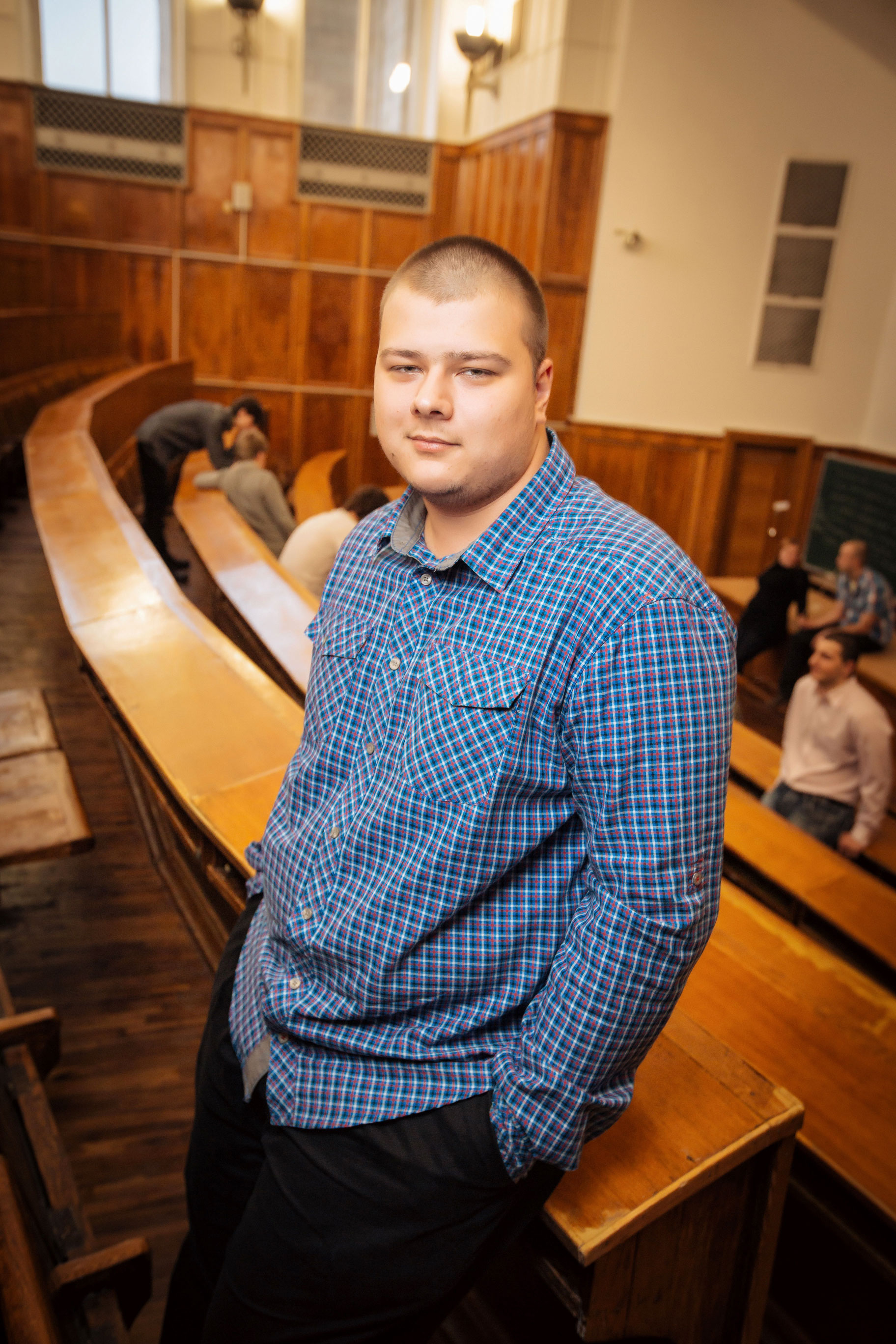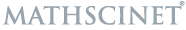﻿ Laboratory site
En / Ru## Kosov Egor Dmitrievich

→ Assistant of the Department of Theory of Functions and Functional Analysis, Faculty of Mechanics and Mathematics, Lomonosov Moscow State University

→ Assistant Professor of the Faculty of Computer Science at the HSE University

E-mail:

Websites:Keywords: logarithmically concave measures, Gaussian measures, measures on infinite dimensional spaces, distributions of polynomials, Nikolskii-Besov classes, discretization of integral norms.

Education:

→ 2006-2009 – high school no. 54 (under the Faculty of Mechanics and Mathematics of Lomonosov Moscow State University);

→ 2009-2014 – Faculty of Mechanics and Mathematics, Lomonosov Moscow State University;

→ 2014-2018 – graduate school of the Department of Theory of Functions and Functional Analysis, Faculty of Mechanics and Mathematics, Lomonosov Moscow State University, advisor Prof. V.I. Bogachev;

→ 2018 – PhD Thesis "Polynomial images and shifts of measures on linear spaces" (Faculty of Mechanics and Mathematics, Lomonosov Moscow State University).

### Research interests:

In laboratory, I work on the problems related to the discretization of integral norms. Besides, my topics of interests are the properties of the regularity of distributions, in particular, the question if the densities of distributions belong to Nikolskii-Besov spaces.

### Teaching:

→ Seminars on mathematical, real and functional analysis for students of the Faculty of Mechanics and Mathematics of MSU.

→ Lectures and seminars on mathematical analysis and probability theory for students of the Faculty of Computer Science at the HSE University.

### Publications (recent):

2023

→ V. I. Bogachev, E. D. Kosov, A. V. Shaposhnikov, "Regularity of solutions to Kolmogorov equations with perturbed drifts", Potential Anal., 58 (2023), 681–702, DOI, arXiv.

2022

→ E. D. Kosov, "Remarks on sampling discretization of integral norms of functions", Approximation Theory, Functional Analysis, and Applications. Collected papers. On the occasion of the 70th birthday of Academician B. S. Kashin. Trudy MIAN, 319 (2022), 202–212, DOI.

→ E. D. Kosov, "Distributions of Second Order Polynomials in Gaussian Random Variables", Math. Notes, 111:1 (2022), 71–81, DOI.

→ B. Kashin, E. Kosov, I. Limonova, V. Temlyakov, "Sampling discretization and related problems", Journal of Complexity, 71(2022), 101653-55, DOI, arXiv.

→ E. Kosov, "Regularity of linear and polynomial images of Skorohod differentiable measures", Advances in Mathematics, 397 (2022), 108193, DOI, arXiv.

→ E. D. Kosov, "Distributions of polynomials in Gaussian random variables under constraints on the powers of variables", Funktsional. Anal. i Prilozhen., 56:2 (2022), 29–38, DOI, arXiv.

2021

→ E. Kosov, "Marcinkiewicz-type discretization of $$L_p$$-norms under the Nikolskii-type inequality assumption", Journal of Mathematical Analysis and Applications, 504:1 (2021), 125358, DOI, arXiv.

→ E. D. Kosov, "Total Variation Distance Estimates via $$L_2$$-Norm for Polynomials in Log-concave Random Vectors", International Mathematics Research Notices, 2021:21 (2021), 16492–16508, DOI, arXiv.

→ V. I. Bogachev, E. D. Kosov, S. N. Popova, "On distributions of homogeneous and convex functions in Gaussian random variables", Izv. Math., 85:5 (2021), 852–882, DOI.

2020

→ V. I. Bogachev, E. D. Kosov, S. N. Popova, "Densities of Distributions of Homogeneous Functions of Gaussian Random Vectors", Doklady Mathematics, 102:3 (2020), 460–463, DOI.

2019

→ E. D. Kosov, "Besov classes on finite and infinite dimensional spaces", Sb. Math., 210:5 (2019), 663–692, DOI, arXiv.

→ V. I. Bogachev, E. D. Kosov, S. N. Popova, "A new approach to Nikolskii-Besov classes", Moscow Mathematical Journal, 19:4 (2019), 619–654, DOI, arXiv.

→ E. D. Kosov, "An Inequality between Total Variation and $$L^2$$ Distances for Polynomials in log-Concave Random Vectors", Doklady Mathematics, 100:2 (2019), 423–425, DOI, arXiv.

→ E. D. Kosov, "On fractional regularity of distributions of functions in gaussian random variables", Fractional Calculus and Applied Analysis, 22:5 (2019), 1249–1268, DOI, arXiv.

2018

→ E. D. Kosov, "Besov Classes on a Space with a Gaussian Measure", Doklady Mathematics, 97:1 (2018), 20–22, DOI.

→ L. Arutyunyan, E. Kosov, "Deviation of polynomials from their expectations and isoperimetry", Bernoulli, 24:3 (2018), 2043–2063, DOI, arXiv.

→ E. D. Kosov, "Fractional smoothness of images of logarithmically concave measures under polynomials", Journal of Mathematical Analysis and Applications, 462:1 (2018), 390–406, DOI, arXiv.

→ V. I. Bogachev, E. D. Kosov, G. I. Zelenov, "Fractional smoothness of distributions of polynomials and a fractional analog of the Hardy-Landau-Littlewood inequality", Transactions of the American Mathematical Society, 370:6 (2018), 4401-4432, DOI, arXiv.

2017

→ V. I. Bogachev, E. D. Kosov, S. N. Popova, "A Characterization of Nikolskii–Besov Classes via Integration by Parts", Doklady Mathematics, 96:2 (2017), 449–453, DOI.

→ V. I. Bogachev, E. D. Kosov, S. N. Popova, "On Gaussian Nikolskii–Besov classes", Doklady Mathematics, 96:2 (2017), 498–502, DOI.

→ E. D. Kosov, "A Characterization of Besov Classes in Terms of a New Modulus of Continuity", Doklady Mathematics, 96:3 (2017), 587–590, DOI.

→ A. V. Kolesnikov, E. D. Kosov, "Moment measures and stability for Gaussian inequalities", Theory Stoch. Process., 22(38):2 (2017), 47–61, PDF, arXiv.